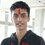# Geometrical Proof

Here is an interesting geometry problem-

Let $A_1$, $A_2 \ \cdots \ A_n$ be the vertices of a regular $n$-sided polygon $P$. Let $X$ be a random point on the incircle of $P$.

Prove that $\displaystyle\sum_{i=1}^{n} \overline{A_iX}^2=nR^2\left[1+\cos^2\left(\dfrac{\pi}{n}\right)\right]$.

Note : Here, $R$ denotes the circumradius of $P$.Note by Pratik Shastri
6 years, 8 months ago

This discussion board is a place to discuss our Daily Challenges and the math and science related to those challenges. Explanations are more than just a solution — they should explain the steps and thinking strategies that you used to obtain the solution. Comments should further the discussion of math and science.

When posting on Brilliant:

• Use the emojis to react to an explanation, whether you're congratulating a job well done , or just really confused .
• Ask specific questions about the challenge or the steps in somebody's explanation. Well-posed questions can add a lot to the discussion, but posting "I don't understand!" doesn't help anyone.
• Try to contribute something new to the discussion, whether it is an extension, generalization or other idea related to the challenge.

MarkdownAppears as
*italics* or _italics_ italics
**bold** or __bold__ bold
- bulleted- list
• bulleted
• list
1. numbered2. list
1. numbered
2. list
Note: you must add a full line of space before and after lists for them to show up correctly
paragraph 1paragraph 2

paragraph 1

paragraph 2

[example link](https://brilliant.org)example link
> This is a quote
This is a quote
    # I indented these lines
# 4 spaces, and now they show
# up as a code block.

print "hello world"
# I indented these lines
# 4 spaces, and now they show
# up as a code block.

print "hello world"
MathAppears as
Remember to wrap math in $$ ... $$ or $ ... $ to ensure proper formatting.
2 \times 3 $2 \times 3$
2^{34} $2^{34}$
a_{i-1} $a_{i-1}$
\frac{2}{3} $\frac{2}{3}$
\sqrt{2} $\sqrt{2}$
\sum_{i=1}^3 $\sum_{i=1}^3$
\sin \theta $\sin \theta$
\boxed{123} $\boxed{123}$

Sort by:

@KARAN SHEKHAWAT Here is the solution :

Let $O$ represent the center of the polygon.

Now, \begin{aligned}\vec{XA_i}&=\vec{XO}+\vec{OA_i}\\ |\vec{XA_i}|^2&=|\vec{XO}|^2+|\vec{OA_i}|^2+2(\vec{XO} \cdot \vec{OA_i})\\ \sum_{i=1}^{n} |\vec{XA_i}|^2&=n(r^2+R^2)+2\sum_{i=1}^{n}(\vec{XO} \cdot \vec{OA_i})\\ &=n(r^2+R^2)+2\vec{XO} \cdot \left(\sum_{i=1}^{n} \vec{OA_i}\right)\end{aligned} Since $\displaystyle\sum_{i=1}^{n} \vec{OA_i}=0$, $\sum_{i=1}^{n} |\vec{XA_i}|^2=\sum_{i=1}^{n} \overline{A_iX}^2=n(R^2+r^2)$

All that's left to do is to express $r$ in terms of $R$. That's a matter of simple trigonometry.

Note 1 : $r$ is the inradius of $P$.

Note 2 : $\displaystyle\sum_{i=1}^{n}(\vec{XO} \cdot \vec{OA_i})=\vec{XO} \cdot \left(\sum_{i=1}^{n} \vec{OA_i}\right)$ due to the distributivity of the dot product over vector addition.

- 6 years, 7 months ago

Awesome ! Thanks a lot ! It's is much better than complex number tecnique atleast for me :) You are genius !

- 6 years, 7 months ago

Can we use particular case of polygon in which vertex are n'th roots of of unity To prove it ?

- 6 years, 8 months ago

You could do that. We are to prove the general result which can also be, to my knowledge, proven that way.

- 6 years, 8 months ago

I'm get stuck in that ! If you Don't mind Can you please Upload its solution So that we can learn from it ?

Thanks !

- 6 years, 8 months ago

Well, I didn't solve it using complex numbers, I used vectors.

- 6 years, 8 months ago

Anyways I will upload it within a day or two.

- 6 years, 8 months ago